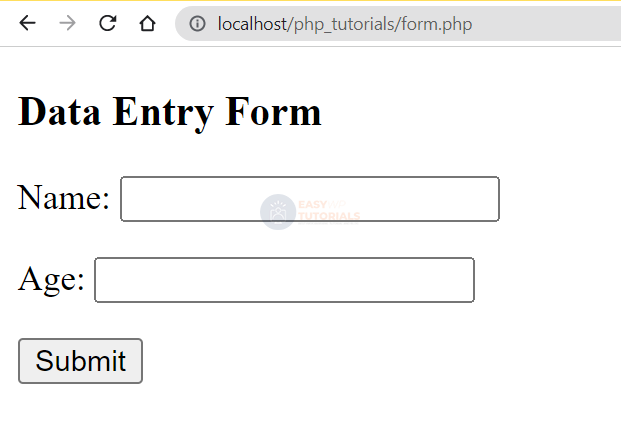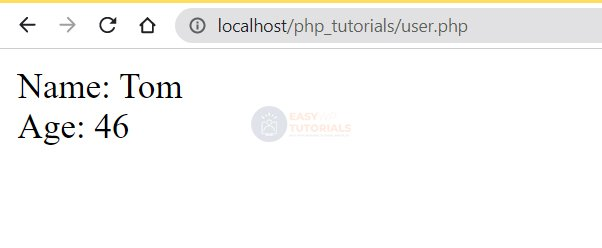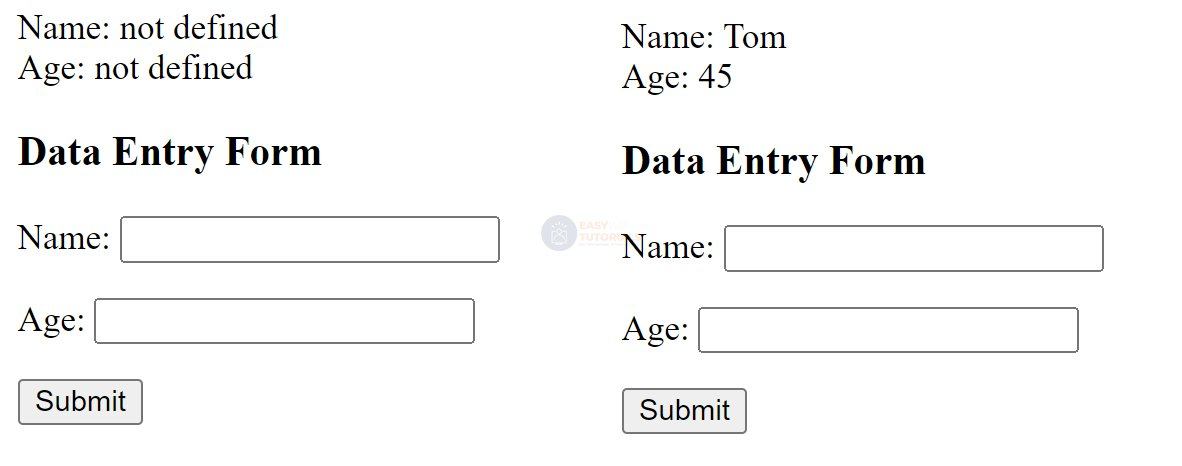# Submitting Forms In PHP

One of the main ways in which data is transmitted to a website is through the processing of forms. Forms are special HTML markup elements that contain various input elements – text fields, buttons, and so on. And with the help of form data, we can enter some data and send it to the server. And the server is already processing this data.

Creating forms consist of the following aspects:

Creating an element <form><form> in HTML markup

Adding one or more input fields to this element

Set the communication method. The most commonly used methods GETarePOST

Setting the address to which the entered data will be sent

POST requests

So let’s create a new form. To do this, we will define a new form.php file , in which we will place the following content:

```<!DOCTYPE html>
<html lang="en">
<meta charset="UTF-8">
<meta http-equiv="X-UA-Compatible" content="IE=edge">
<meta name="viewport" content="width=device-width, initial-scale=1.0">
<title>Data Entry Form :: easywptutorials.com</title>
<body>
<h3>Data Entry Form</h3>
<form action="user.php" method="POST">
<p>Name: <input type="text" name="name" /></p>
<p>Age: <input type="number" name="age" /></p>
<input type="submit" value="Submit">
</form>
</body>
</html>
```

action=”user.php” The element attribute form indicates that the form data will be processed by the user.php script, which will be located with the file form.php in the same folder. And the attribute method=”POST” indicates that the POST method will be used as the data transfer method.

Now let’s define a file user.php that will have the following content:

```<?php
\$name = "not defined";
\$age = "not defined";
if(isset(\$_POST["name"])){

\$name = \$_POST["name"];
}
if(isset(\$_POST["age"])){

\$age = \$_POST["age"];
}
echo "Name: \$name <br> Age: \$age";
?>
```

PHP uses the \$_POST built-in global variable to handle POST requests. It represents an associative array of data submitted using the POST method. Using the keys, we can get the submitted values. The keys in this array are the attribute values ​​of name the form input fields.

For example, since the attribute name of the age input field has a value age(<input type=”number” name=”age” />), then in the array \$_POST, the value of this field will represent the key “age”:\$_POST[“age”]

And since there are situations when the input field is not set, in this case it is desirable to check their presence using the isset() function before processing the data. And if the variable is set, then the isset() function will return the value true.

Now we can access the form.php script and enter some data into the form:And when the button is clicked, the entered data will be sent to the user.php script using the POST method :It is not necessary to send the form data to another script, it is possible to process the form data in the same form file. To do this, change the form.php file as follows:

```<!DOCTYPE html>
<html lang="en">
<metacharset="UTF-8">
<meta http-equiv="X-UA-Compatible" content="IE=edge">
<meta name="viewport" content="width=device-width, initial-scale=1.0">
<title>Data Entry Form :: easywptutorials.com</title>
<body>
<?php
\$name = "not defined";
\$age = "not defined";
if(isset(\$_POST["name"])){

\$name = \$_POST["name"];
}
if(isset(\$_POST["age"])){

\$age = \$_POST["age"];
}
echo "Name: \$name <br> Age: \$age";
?>
<h3>Data Entry Form</h3>
<form method="POST">
<p>Name: <input type="text" name="name" /></p>
<p>Age: <input type="number" name="age" /></p>
<input type="submit" value="Submit">
</form>
</body>
</html>
```

Since in this case we are sending data to the same script – that is, to the same address, the form element does not need to have the action.It is worth noting that, in principle, we can send forms with a GET request, in this case, the \$_GET array , which was discussed in the previous topic, is used to get the same form values:

```<!DOCTYPE html>
<html lang="en">
<metacharset="UTF-8">
<meta http-equiv="X-UA-Compatible" content="IE=edge">
<meta name="viewport" content="width=device-width, initial-scale=1.0">
<title>Data Entry Form :: easywptutorials.com</title>
<body>
<?php
\$name = "not defined";
\$age = "not defined";
if(isset(\$_POST["name"])){

\$name = \$_POST["name"];
}
if(isset(\$_POST["age"])){

\$age = \$_POST["age"];
}
echo "Name: \$name <br> Age: \$age";
?>
<h3>Data Entry Form</h3>
<form method="POST">
<p>Name: <input type="text" name="name" /></p>
<p>Age: <input type="number" name="age" /></p>
<input type="submit" value="Submit">
</form>
</body>
</html>
```